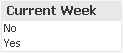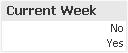# QlikView Documents

Documents for QlikView related information.

# Customized flagsEmployee

## Customized flags

I work a lot with flags in my data. This makes it easier to interpret selections and define set expressions. This example shows how and why I customize the flags during load to make my data and expressions even clearer and more intuitive.

A flag typically has two states, which correspond to true or false. The flag answers a logical comparison with a true or false, for example the flag could indicate if a date is in current week. The logical comparison is if MyDate has the week number as Today.

```If( Week(MyDate) = Week(Today()), True(), False())
```

In QlikView true is -1 and false is 0, when ever the values are calculated from comparison or called from the boolean functions. The actual definition is that zero is false, and all other values are true. For this reason it is common that flags are set to 1 or 0.

```If( Week(MyDate) = Week(Today()), 1, 0)
```

The down side with this is that if the flag field is use din selections the list looks quite dull, and is not very intuitive for an end-user.To make sure the end-user understands what the values represent, the list is set up with an expression to mask the values.

```If(IsCurrentWeek, 'Yes', 'No')
```

This leads to some manual labor if the application has multiple flags, and if the source data is reused in multiple apps. But, the user interface is now a bit clearer.A much better way of doing this is to only define the value once. The place to define the data is in the script. The first thing to do is to define the Yes and No values. Notice that in this example I do this by using SET and defining two Dual() definitions. The text value can be customized for what ever the flag represents.

```SET cYES = Dual('Yes', 1);
SET cNO = Dual('No', 0);
```

The comparison is done the same way as before. Instead of the 1 and 0, the Yes and No variables are expanded into the expression.

```If( Week(MyDate) = Week(Today()), \$(cYES), \$(cNO))
```

In a list box the field can now be displayed directly. Compared to the list box above, the text is right aligned which indicates that is has an underlying numerical value. This means that the field can successfully be used in aggregations and comparisons.In the attached example I have applied the above principle for flagging calendar ranges. This makes set expressions easy to read as it now has a text based set modifier instead of a numerical flag.

```count({<Is30DayPeriod={"Yes"}, WeekDay={"Mon"}>} Date)
```

The flag can still be used in aggregations since it has the underlying numerical values.

```sum(IsLeftCurrentYear)
```

This is an extension of my Calendar with period flags‌ example.

Labels (4)

• ### Scripting

Version history
Revision #:
1 of 1
Last update:
‎2015-05-14 08:10 AM
Updated by: